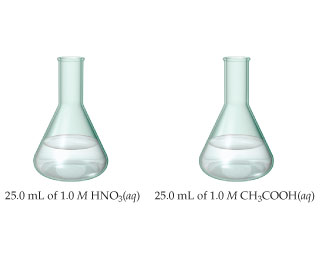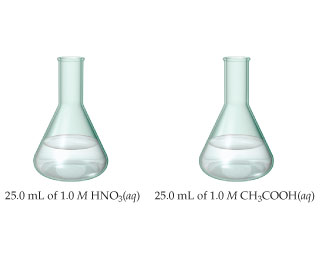# Problem: The samples of nitric and acetic acid shown here are both titrated with a 0.100 M solution of NaOH(aq).Determine whether each of the following statements concerning these titrations is true or false.The pH at the equivalence point in the HNO3 titration will be lower than the pH at the equivalence point in the CH3COOH titration.

###### FREE Expert Solution

We are asked if the pH at the equivalence point in the HNO3 titration will be lower than the pH at the equivalence point in the CH3COOH titration where both were titrated with a 0.100 M solution of NaOH(aq).1. Titration of 25.0 mL of 1.0 M HNO3 with 0.100 M NaOH solution

The reaction for this titration can be represented by:

HNO3 + NaOH → NaNO3 + H2O

In the reaction HNO3, a strong acid, will react with NaOH, a strong base to form a neutral solution.

At the equivalence point of the reaction, hydronium (H+) and hydroxide (OH-) ions will react to form water, leading to a pH of 7.

This is true for all strong acid and strong base reactions.

2. Titration of 25 mL of 1.0 M CH3COOH with 0.100 M NaOH

This reaction is a Weak Acid – Strong Base titration.

The reaction between HCH3COO and NaOH will be a double displacement reaction where H+ reacts with OH and CH3COO reacts with Na+

The overall reaction is:

HCH3COO + NaOHH2O + NaCH3COO

Recall that at the equivalence point of a titration:

79% (478 ratings)###### Problem Details

The samples of nitric and acetic acid shown here are both titrated with a 0.100 M solution of NaOH(aq).
Determine whether each of the following statements concerning these titrations is true or false.The pH at the equivalence point in the HNO3 titration will be lower than the pH at the equivalence point in the CH3COOH titration.